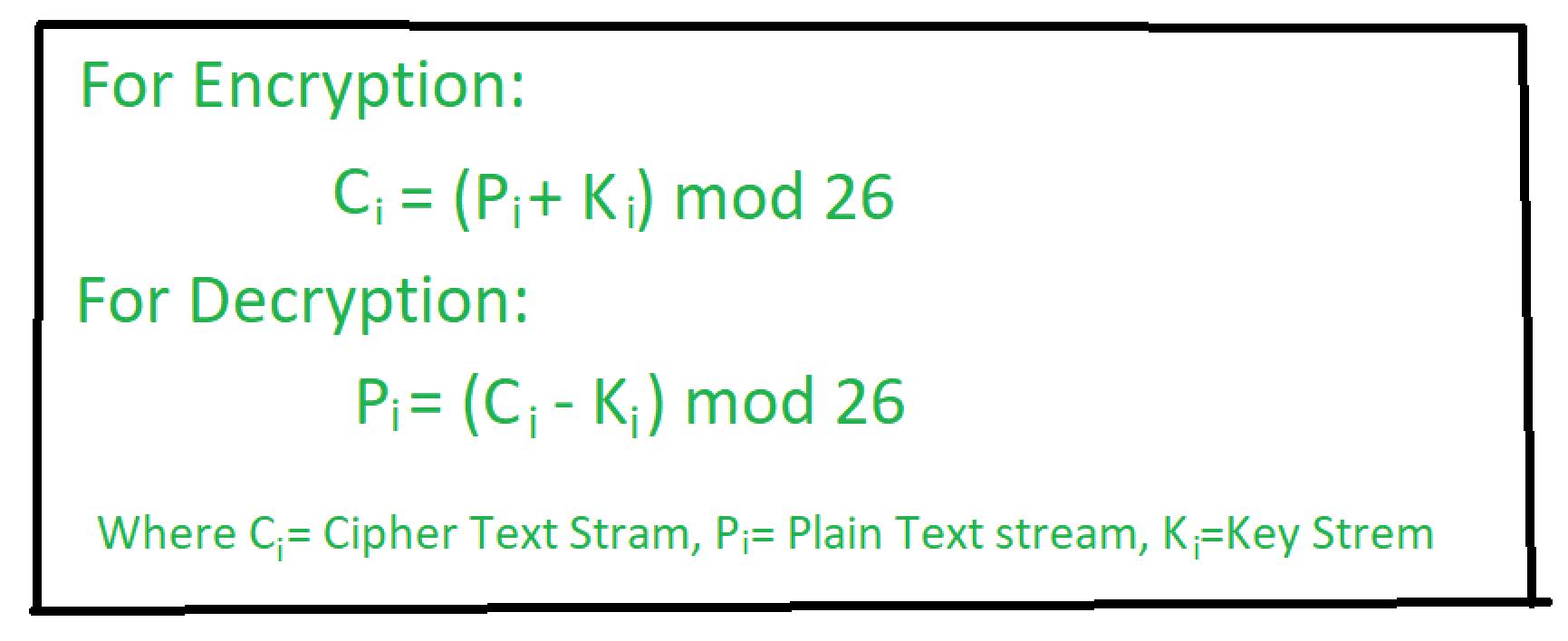# Autokey Cipher | Symmetric Ciphers

• Difficulty Level : Medium
• Last Updated : 10 May, 2020

Autokey Cipher is a polyalphabetic substitution cipher. It is closely related to the Vigenere cipher but uses a different method of generating the key. It was invented by Blaise de Vigenère in 1586. In general, more secure than the Vigenere cipher.Example-1:

```Plaintext = "HELLO"
Autokey = N
Ciphertext = "ULPWZ" ```

Example-2:

```Plaintext = "GEEKSFORGEEKS"
Autokey = P
Ciphertext = "VKIOCXTFXKIOC" ```

In this cipher, the key is a stream of subkeys which is used to encrypt the corresponding character in the plaintext.As shown, the autokey is added at the first of the subkeys.

```Let's explain Example 1:

Given plain text is : H E L L O
Key is              : N H E L L

Let's encrypt:

Plain Text(P)       : H   E   L   L   O
Corresponding Number: 7   4   11  11  14
Key(K)              : N   H   E   L   L
Corresponding Number: 13  7   4   11  11
---------------------
Applying the formula: 20  11  15  22  25

Corresponding
Letters are         : U    L   P   W   Z

Hence Ciphertext is: ULPWZ

Let's decrypt:

Cipher Text(C)      : U   L   P   W   Z
Key(K)              : N   H   E   L   L
---------------------
Applying the formula: H   E   L   L   O

Hence Plaintext is: HELLO ```

Here’s the java code for Autokey Cipher.

## Java

 `// A JAVA program to illustrate``// Autokey Cipher Technique`` ` `// Importing required library``import` `java.lang.*;``import` `java.util.*;`` ` `public` `class` `AutoKey {`` ` `    ``private` `static` `final` `String alphabet = ``"ABCDEFGHIJKLMNOPQRSTUVWXYZ"``;`` ` `    ``public` `static` `void` `main(String[] args)``    ``{``        ``String msg = ``"HELLO"``;``        ``String key = ``"N"``;`` ` `        ``// This if statement is all about java regular expression``        ``// [] for range``        ``// // Extra \ is used to escape one \``        ``// \\d acts as delimiter``        ``// ? once or not at all``        ``// . Any character (may or may not match line terminators)``        ``if` `(key.matches(``"[-+]?\\d*\\.?\\d+"``))``            ``key = ``""` `+ alphabet.charAt(Integer.parseInt(key));``        ``String enc = autoEncryption(msg, key);`` ` `        ``System.out.println(``"Plaintext : "` `+ msg);``        ``System.out.println(``"Encrypted : "` `+ enc);``        ``System.out.println(``"Decrypted : "` `+ autoDecryption(enc, key));``    ``}`` ` `    ``public` `static` `String autoEncryption(String msg, String key)``    ``{``        ``int` `len = msg.length();`` ` `        ``// generating the keystream``        ``String newKey = key.concat(msg);``        ``newKey = newKey.substring(``0``, newKey.length() - key.length());``        ``String encryptMsg = ``""``;`` ` `        ``// applying encryption algorithm``        ``for` `(``int` `x = ``0``; x < len; x++) {``            ``int` `first = alphabet.indexOf(msg.charAt(x));``            ``int` `second = alphabet.indexOf(newKey.charAt(x));``            ``int` `total = (first + second) % ``26``;``            ``encryptMsg += alphabet.charAt(total);``        ``}``        ``return` `encryptMsg;``    ``}`` ` `    ``public` `static` `String autoDecryption(String msg, String key)``    ``{``        ``String currentKey = key;``        ``String decryptMsg = ``""``;`` ` `        ``// applying decryption algorithm``        ``for` `(``int` `x = ``0``; x < msg.length(); x++) {``            ``int` `get1 = alphabet.indexOf(msg.charAt(x));``            ``int` `get2 = alphabet.indexOf(currentKey.charAt(x));``            ``int` `total = (get1 - get2) % ``26``;``            ``total = (total < ``0``) ? total + ``26` `: total;``            ``decryptMsg += alphabet.charAt(total);``            ``currentKey += alphabet.charAt(total);``        ``}``        ``return` `decryptMsg;``    ``}``}`

```Output:

Plaintext : HELLO
Encrypted : ULPWZ
Decrypted : HELLO

```

My Personal Notes arrow_drop_up